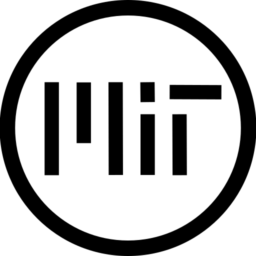# 2D Pixelart Upscaler/Filter

Shader for upscaling pixelart. Works with Godot 4.

Important the image has to be filtered.

``````shader_type canvas_item;

uniform float FILTER_GAMMA = 1.0;
uniform float COLOR_GAMMA = 1.0;

vec2 snap_UV1(vec2 uv, vec2 steps) {
return (floor(uv / steps) + 0.5) * steps;
}

float length_squared(vec4 v0) {
return v0.x*v0.x + v0.y*v0.y + v0.z*v0.z + v0.w*v0.w ;
}
void fragment() {
vec2 shift = vec2(-TEXTURE_PIXEL_SIZE *.5);
vec2 pixel_size = TEXTURE_PIXEL_SIZE;

// sample the color from the filtered image
vec4 color_sample0 = texture(TEXTURE, UV + pixel_size * .5 + shift);

// sample the color from 4 points at positions with a small influence of interpolation
vec2 sample_uv = snap_UV1(UV + shift, pixel_size);
vec2 offset = pixel_size;
vec4 color_sample1 = texture(TEXTURE, sample_uv + vec2(0.0,0.0));
vec4 color_sample2 = texture(TEXTURE, sample_uv + vec2(+offset.x,0.0));
vec4 color_sample3 = texture(TEXTURE, sample_uv + vec2(0.0,+offset.y));
vec4 color_sample4 = texture(TEXTURE, sample_uv + vec2(+offset.x,+offset.y));

COLOR = color_sample0;

// gamma adjusment for filtering, affects the brightness influence
color_sample0 = pow(color_sample0, vec4(FILTER_GAMMA));
color_sample1 = pow(color_sample1, vec4(FILTER_GAMMA));
color_sample2 = pow(color_sample2, vec4(FILTER_GAMMA));
color_sample3 = pow(color_sample3, vec4(FILTER_GAMMA));
color_sample4 = pow(color_sample4, vec4(FILTER_GAMMA));

// calculating the diviation
float d1 = length_squared(color_sample0 - color_sample1);
float d2 = length_squared(color_sample0 - color_sample2);
float d3 = length_squared(color_sample0 - color_sample3);
float d4 = length_squared(color_sample0 - color_sample4);

float d0 = 1000.0;

COLOR = color_sample0;

if (d0 > d1) {
d0 = d1;
COLOR = color_sample1;
}

if (d0 > d2) {
d0 = d2;
COLOR = color_sample2;
}
if (d0 > d3) {
d0 = d3;
COLOR = color_sample3;
}

if (d0 > d4) {
d0 = d4;
COLOR = color_sample4;
}

COLOR = pow(COLOR, vec4(COLOR_GAMMA/FILTER_GAMMA));
}``````
###### Tags
filter, pixel, pixelart, size, upscalerThe shader code and all code snippets in this post are under MIT license and can be used freely. Images and videos, and assets depicted in those, do not fall under this license. For more info, see our License terms.

## More from Firerabbit

### Sobel with Gaussian filter

Subscribe
Notify of1 Comment
Inline Feedbacks